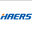# ZheJiang Haers Vacuum Containers Co Ltd SZSE:002615ZheJiang Haers Vacuum Containers Co Ltd
SZSE:002615
Price: 7.05 CNY -3.42%
Updated:

## Discount Rate

### Cost of Equity Discount Rate6.3%
2.68%
0.81
4.47%ZheJiang Haers Vacuum Containers Co Ltd's Cost of Equity, calculated using the formula Risk-Free Rate + Beta x ERP, stands at 6.3%. The Beta, indicating the stock's volatility relative to the market, is 0.81, while the current Risk-Free Rate, based on government bond yields, is 2.68%, and the ERP, measuring the extra return over the risk-free rate required by investors, is 4.47%.

### WACC Discount Rate6.11%
14.53%
5%
6.3%ZheJiang Haers Vacuum Containers Co Ltd's Weighted Average Cost of Capital (WACC) is calculated as the weighted average of its cost of equity and cost of debt, adjusted for tax. The WACC stands at 6.11%. This includes the cost of equity at 6.3%, calculated as Risk-Free Rate + Beta x ERP, and the cost of debt at 5%, reflecting the interest rate on AMZN's debt adjusted for tax benefits. The weight of debt in the capital structure is 14.53%.

## What is ZheJiang Haers Vacuum Containers Co Ltd's discount rate?ZheJiang Haers Vacuum Containers Co Ltd 's current Cost of Equity is 6.3%, while its WACC stands at 6.11%. The selection of the appropriate discount rate is contingent on the type of cash flows being discounted.

For Equity Valuation: When valuing equity, especially in scenarios where you are discounting cash flows to equity holders (such as Net Income, Earnings Per Share (EPS), or Free Cash Flow to Equity), the Cost of Equity should be used.

For Firm Valuation: In contrast, when valuing the entire firm and discounting cash flows available to both debt and equity holders (like Free Cash Flow to the Firm), the Weighted Average Cost of Capital (WACC) is the appropriate rate."

## How is Cost of Equity for ZheJiang Haers Vacuum Containers Co Ltd calculated?

The Cost of Equity represents the return a company must offer investors to compensate for the risk of investing in its stock. It's calculated using the Capital Asset Pricing Model (CAPM), which combines the risk-free rate, the stock's beta, and the equity risk premium (ERP).

This model considers the inherent risk of investing in the stock compared to a risk-free investment and the market's overall risk.

Here is how we calculate the cost of equity forZheJiang Haers Vacuum Containers Co Ltd

Cost of Equity
6.3%
=
Risk-Free Rate
2.68%
+
Beta
0.81
x
ERP
4.47%

## How is WACC for ZheJiang Haers Vacuum Containers Co Ltd calculated?

WACC, or Weighted Average Cost of Capital, is a calculation that reflects the average rate of return a company is expected to pay its security holders to finance its assets. It is a critical measure in financial analysis for valuing a company’s entire operations.

The WACC formula combines the costs of equity and debt, weighted by their respective proportions in the company's capital structure.

Here is how we calculate WACC forZheJiang Haers Vacuum Containers Co Ltd

WACC
6.11%
=
Cost of Equity
6.3%
x
Equity Weight
85%
+
Cost of Debt
5%
x
Debt Weight
15%